In Fall 2015, I took CS 276, a graduate level introduction to theoretical cryptography. For the final project, I gave a short presentation on secure computation.

This blog post is adapted and expanded from my presentation notes. The formal proofs of security rely on a lot of background knowledge, but the core ideas are very clean, and I believe anyone interested in theoretical computer science can understand them.

## What is Secure Computation?

In secure computation, each party has some private data. They would like to learn the answer to a question based off everyone’s data. In voting, this is the majority opinion. In an auction, this is the winning bid. However, they also want to maintain as much privacy as possible. Voters want to keep their votes secret, and bidders don’t want to publicize how much they’re willing to pay.

More formally, there are $$n$$ parties, the $$i$$th party has input $$x_i$$, and everyone wants to know $$f(x_1, x_2, \cdots, x_n)$$ for some function $$f$$. The guiding question of secure computation is this: when can we securely compute functions on private inputs without leaking information about those inputs?

This post will focus on the two party case, showing that every function is securely computable with the right computational assumptions.

## Problem Setting

Following crypto tradition, Alice and Bob are two parties. Alice has private information $$x$$, and Bob has private information $$y$$. Function $$f(x,y)$$ is a public function that both Alice and Bob want to know, but neither Alice nor Bob wants the other party to learn their input.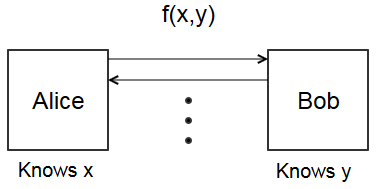The classical example is Yao’s Millionaire Problem. Alice and Bob want to know who has more money. It’s socially unacceptable to brag about your wealth, so neither wants to say how much money they have. Let $$x$$ be Alice’s wealth, and $$y$$ be Bob’s wealth. Securely computing

$f(x,y) = \begin{cases} \text{Alice} & \text{if } x > y \\ \text{Bob} & \text{if } x < y \\ \text{same} & \text{if } x = y \end{cases}$

lets Alice and Bob learn who is richer.

Before going any further, I should explain what security guarantees we’re aiming for. We’re going to make something secure against semi-honest adversaries, also known as honest-but-curious. In the semi-honest model, Alice and Bob never lie, following the specified protocol exactly. However, after the protocol, Alice and Bob will analyze the messages received to try to extract information about the other person’s input.

Assuming neither party lies is naive and doesn’t give a lot of security in real life. However, it’s still difficult to build a protocol that leaks no unnecessary information, even in this easier setting. Often, semi-honest protocols form a stepping stone towards the malicious case, where parties can lie. Explaining protocols that work for malicious adversaries is outside the scope of this post.

## Informal Definitions

To prove a protocol is secure, we first need to define what security means. What makes secure computation hard is that Alice and Bob cannot trust each other. Every message can be analyzed for information later, so they have to be very careful in what they choose to send to one another.

Let’s make the problem easier. Suppose there was a trusted third party named Faith. With Faith, Alice and Bob could compute $$f(x,y)$$ without directly communicating.

• Alice sends $$x$$ to Faith
• Bob sends $$y$$ to Faith
• Faith computes $$f(x,y)$$ and sends the result back to Alice and Bob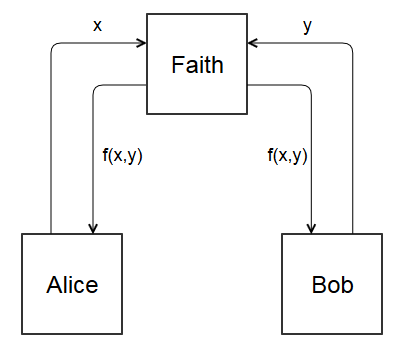This is called the ideal world, because it represents the best case scenario. All communication goes through Faith, who never reveals the input she received. She only replies with what the parties want to know, $$f(x,y)$$.

If this is the best case scenario, we should define security by how close we get to that best case. This gives the following.

A protocol between Alice and Bob is secure if it is as secure as the ideal world protocol between Alice, Bob, and Faith.

To make this more precise, we need to analyze the ideal world’s security.

Suppose we securely computed $$f(x,y) = x+y$$ in the ideal world. At the end of communication, Alice knows $$x$$ and $$x+y$$, while Bob knows $$y$$ and $$x + y$$. If Alice computes

$(x+y) - x$

she learns Bob’s input $$y$$. Similarly, if Bob computes

$(x+y) - y$

he learns Alice’s input $$x$$. Even the ideal world can leak information. In an extreme case like this, possibly even the entire input! If Alice and Bob want to know $$f(x,y) = x + y$$, they have to give up any hope of privacy.

This is an extreme example, but most functions leak something about each party. Going back to the millionaire’s problem, suppose Alice has $$10$$ dollars and Bob has $$8$$ dollars. They securely compute who has the most money, and learn Alice is richer. At this point,

• Alice knows Bob has less than $$10$$ dollars because she’s richer.
• Bob knows Alice has more than $$8$$ dollars because he’s poorer.

However, there is still some privacy: neither knows the exact wealth of the other.

Since $$f(x,y)$$ must be given to Alice and Bob, the best we can do is learn nothing more than what was learnable from $$f(x,y)$$. This gives the final definition.1

A protocol between Alice and Bob is secure if Alice learns only information computable from $$x$$ and $$f(x,y)$$, and Bob learns only information computable from $$y$$ and $$f(x,y)$$

(Side note: if you’ve seen zero-knowledge proofs, you can alternatively think of a protocol as secure if it is simulatable from $$x, f(x,y)$$ for Alice and $$y, f(x,y)$$ for Bob. In fact, this is how you would formally prove security. If you haven’t seen zero-knowledge proofs, don’t worry, they won’t show up in later sections, and we’ll argue security informally.)

Armed with a security definition, we can start constructing the protocol. Before doing so, we’ll need a few cryptographic assumptions.

## Cryptographic Primitives

All of theoretical cryptography rests on assuming some problems are harder than other problems. These problems form the cryptographic primitives, which can be used for constructing protocols.

For example, one cryptographic primitive is the one-way function. Abbreviated OWF, these functions are easy to compute, but hard to invert, where easy means “doable in polynomial time” and hard means “not doable in polynomial time.” Assuming OWFs exist, we can create secure encryption, pseudorandom number generators, and many other useful things.

No one has proved one-way functions exist, because proving that would prove $$P \neq NP$$, and that’s, well, a really hard problem, to put it mildly. However, there are several functions that people believe to be one-way, which can be used in practice.

For secure computation, we need two primitives: oblivious transfer, and symmetric encryption.

# Oblivious Transfer

Oblivious transfer (abbreviated OT) is a special case of secure computation. Alice has two messages $$m_0, m_1$$. Bob has a bit $$b$$. We treat oblivious transfer as a black box method where

• Alice gives $$m_0, m_1$$
• Bob gives bit $$b$$, $$0$$ or $$1$$
• If $$b = 0$$, Bob gets $$m_0$$. Otherwise, he gets $$m_1$$. In both cases, Bob does not learn the other message.
• Alice does not learn which message Bob received. She only knows Bob got one of them.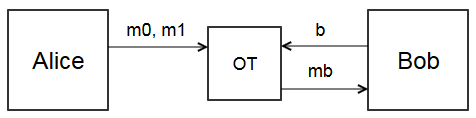You can think of the OT box as a trusted third party, like Faith. Letting Alice send messages without knowing which message was received will be key to the secure computation protocol. I don’t want to get into the details of implementing OT with only Alice and Bob, since they aren’t that interesting. If you’re curious, Wikipedia has a good example based on RSA.

# Symmetric Encryption

Symmetric encryption allows two people to send encrypted messages that cannot be decrypted without the secret key. Formally, a symmetric encryption scheme is a pair of functions, encrypter $$E$$ and decrypter $$D$$, such that

• $$E_k(m)$$ is the encryption of message $$m$$ with key $$k$$.
• $$D_k(c)$$ is the decryption of ciphertext $$c$$ with key $$k$$.
• Decrypting with the same secret key gives the original message, or $$D_k(E_k(m)) = m$$,
• Given just ciphertext $$c$$, it is hard to find a key $$k$$ and message $$m$$ such that $$E_k(m) = c$$. (Where again, hard means not solvable in polynomial time.)

The above is part of the standard definition for symmetric encryption. For the proof, we’ll need one more property that’s less conventional.

• Decrypting $$E_k(m)$$ with a different key $$k'$$ results in an error.

With all that out of the way, we can finally start talking about the protocol itself.

## Yao’s Garbled Circuits

Secure computation was first formally studied by Andrew Yao in the early 1980s. His introductory paper demonstrated protocols for a few examples, but did not prove every function was securely computable. That didn’t happen until 1986, with the construction of Yao’s garbled circuits.

To prove every function was securely computable, Yao proved every circuit was securely computable. Every function can be converted to an equivalent circuit, and we’ll see that working in the circuit model of computation makes the construction simpler.

# A Quick Circuit Primer

For any function $$f(x,y)$$, there is some circuit $$C$$ such that $$C(x,y)$$ gives the same output. That circuit $$C$$ is made up of logic gates and wires connecting them. Each logic gate has two input wires and one output wire, and computes a simple Boolean function, like AND or OR.

The wires in a circuit can be divided into three categories: inputs for the circuit, outputs for the circuit, and intermediate wires between gates.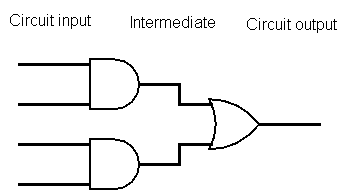# Garbling Circuits

Here is a very obviously insecure protocol for computing $$f(x,y)$$

• Alice sends circuit $$C$$ to Bob.
• Alice sends her input $$x$$ to Bob.
• Bob evaluates the circuit to get $$f(x,y)$$
• Bob sends $$f(x,y)$$ back to Alice.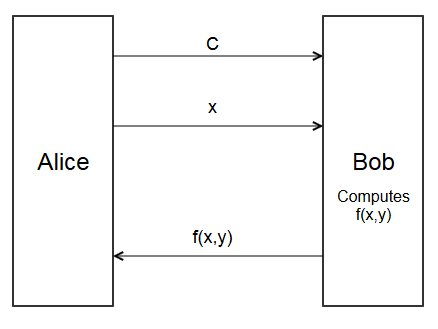This works, but Alice has to send $$x$$ to Bob.

This is where garbling comes in. Instead of sending $$C$$, Alice will send a garbled circuit $$G(C)$$. Instead of sending $$x$$, she’ll send $$x$$ encoded in a certain way. It will be done such that Bob can evaluate $$G(C)$$ with Alice’s encoded input, without learning what Alice’s original input $$x$$ was, and without exposing any wire values except for the final output.2

Number the wires of the circuit as $$w_1, w_2, \ldots, w_n$$. For each wire $$w_i$$, generate two random encryption keys $$k_{i,0}$$ and $$k_{i,1}$$. These will represent $$0$$ and $$1$$.

A garbled circuit is made of garbled logic gates. Garbled gates act the same as regular logic gates, except they operate on the sampled encryption keys instead of bits.

Suppose we want to garble an OR gate. It has input wires $$w_1,w_2$$, and output wire $$w_3$$.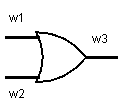Internally, an OR gate is implemented with transistors. However, at our level of abstraction we treat the OR gate as a black box. The only thing we care about is that it follows this behavior.

• Given $$w_1 = 0, w_2 = 0$$, it sets $$w_3 = 0$$.
• Given $$w_1 = 0, w_2 = 1$$, it sets $$w_3 = 1$$.
• Given $$w_1 = 1, w_2 = 0$$, it sets $$w_3 = 1$$.
• Given $$w_1 = 1, w_2 = 1$$, it sets $$w_3 = 1$$.

This is summarized in the function table below.

$\begin{array}{ccc} w_1 & w_2 & w_3 \\ 0 & 0 & 0 \\ 0 & 1 & 1 \\ 1 & 0 & 1 \\ 1 & 1 & 1 \end{array}$

A garbled OR gate goes one step further.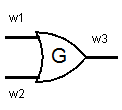It instead takes $$k_{1,0}, k_{1,1}$$ for $$w_1$$, and $$k_{2,0}, k_{2,1}$$ for $$w_2$$. Treating these keys as bits $$0$$ or $$1$$, the output is $$k_{3,0}$$ or $$k_{3,1}$$, depending on which bit the gate is supposed to return. You can think of it as replacing the OR gate with a black box that acts as follows.

• Given $$w_1 = k_{1,0}, w_2 = k_{2,0}$$, it sets $$w_3 = k_{3,0}$$.
• Given $$w_1 = k_{1,0}, w_2 = k_{2,1}$$, it sets $$w_3 = k_{3,1}$$.
• Given $$w_1 = k_{1,1}, w_2 = k_{2,0}$$, it sets $$w_3 = k_{3,1}$$.
• Given $$w_1 = k_{1,1}, w_2 = k_{2,1}$$, it sets $$w_3 = k_{3,1}$$.
$\begin{array}{ccc} w_1 & w_2 & w_3 \\ k_{1,0} & k_{2,0} & k_{3,0} \\ k_{1,0} & k_{2,1} & k_{3,1} \\ k_{1,1} & k_{2,0} & k_{3,1} \\ k_{1,1} & k_{2,1} & k_{3,1} \end{array}$

By replacing every logic gate with a garbled gate, we can evaluate the circuit using random encryption keys instead of bits.

We implement this using symmetric encryption. For every possible pair of input keys, the correct output is encrypted using both those keys. Doing this for all possible inputs gives the garbled table,

$\begin{array}{c} E_{k_{1,0}}(E_{k_{2,0}}(k_{3,0})) \\ E_{k_{1,0}}(E_{k_{2,1}}(k_{3,1})) \\ E_{k_{1,1}}(E_{k_{2,0}}(k_{3,1})) \\ E_{k_{1,1}}(E_{k_{2,1}}(k_{3,1})) \end{array}$

With one secret key for each wire, Bob can get the correct output by attempting to decrypt all 4 values. Suppose Bob has $$k_{1,0}$$ and $$k_{2,1}$$. Bob first decrypts with $$k_{1,0}$$. He’ll decrypt exactly two values, and will get an error on the other two.

$\begin{array}{c} E_{k_{2,0}}(k_{3,0}) \\ E_{k_{2,1}}(k_{3,1}) \\ \text{error} \\ \text{error} \end{array}$

Decrypting the two remaining messages with $$k_{2,1}$$ gives

$\begin{array}{c} \text{error} \\ k_{3,1} \\ \text{error} \\ \text{error} \end{array}$

getting the key corresponding to output bit $$1$$ for wire $$w_3$$.

(To be fully correct, we also shuffle the garbled table, to make sure the position of the decrypted message doesn’t leak anything.)

Creating the garbled table for every logic gate in the circuit gives the garbled circuit. Informally, here’s why the garbled circuit can be evaluated securely.

• For a given garbled gate, Bob cannot read any value in the garbled table unless he has a secret key for each input wire, because the values are encrypted.
• Starting with the keys for the circuit input, Bob can evaluate the garbled gates in turn. Each garbled gate returns exactly one key, and that key represents exactly the correct output bit. When Bob gets to the output wires, he must get the same output as the original circuit.
• Both $$k_{i,0}$$ and $$k_{i,1}$$ are generated randomly. Given just one of them, Bob has no way to tell whether the key he has represents $$0$$ or $$1$$. (Alice knows, but Alice isn’t the one evaluating the circuit.)

Thus, from Bob’s perspective, he’s evaluating the circuit by passing gibberish as input, propagating gibberish through the garbled gates, and getting gibberish out. He’s still doing the computation - he just has no idea what bits he’s actually looking at.

(The one minor detail left is key generation for the output wires of the circuit. Instead of generating random keys, Alice uses the raw bit $$0$$ or $$1$$, since Alice needs Bob to learn the circuit output.)

# The Last Step

Here’s the new protocol, with garbled circuits

• Alice garbles circuit $$C$$ to get garbled circuit $$G(C)$$
• Alice sends $$G(C)$$ to Bob.
• Alice sends the keys for her input $$x$$ to Bob.
• Bob combines them with the input keys for $$y$$, and evaluates $$G(C)$$ to get $$f(x,y)$$
• Bob sends $$f(x,y)$$ back to Alice.There’s still a problem. How does Bob get the input keys for $$y$$? Only Alice knows the keys created for each wire. Bob could give Alice his input $$y$$ to get the right keys, but then Alice would learn $$y$$.

One solution is to have Alice send both keys for each of $$y$$’s input wires to Bob. For each wire, Bob can then pick the key corresponding to the correct bit of $$y$$. That way, Bob doesn’t have to give Alice $$y$$, but can still run the circuit.

However, this has a subtle bug: by giving Bob both keys for his input wires, Alice lets Bob run the circuit with an arbitrary $$y$$. Letting Bob evaluate $$f(x,y)$$ many times gives Bob more information. Going back to the millionaire’s problem, let $$x = 10$$ and $$y = 8$$. At the end, Bob learns Alice is richer, meaning $$x > 8$$. But, if he later evaluates $$f(x, 9)$$, he’ll learn $$x > 9$$, which is more than he should know.

Thus, to be secure, Bob should only get to run the circuit on exactly $$x,y$$. To do so, he needs to get the keys for $$y$$, and only the keys for $$y$$, without Alice learning which keys he wants. If only there was a way for Alice to obliviously transfer those keys…

Alright, yes, that’s why we need oblivious transfer. Using oblivious transfer to fill in the gap, we get the final protocol.

• Alice garbles circuit $$C$$ to get garbled circuit $$G(C)$$
• Alice sends $$G(C)$$ to Bob.
• Alice sends the keys for her input $$x$$ to Bob.
• Using oblivious transfer, for each of Bob’s input wires, Alice sends $$k_{i,y_i}$$ to Bob.
• With all input keys, Bob can evaluate the circuit to get $$f(x,y)$$
• Bob sends $$f(x,y)$$ back to Alice.This lets Alice and Bob compute $$f(x,y)$$ without leaking their information, and without trusted third parties.

## Conclusion

This protocol is mostly a theoretical exercise. However, there has been recent work to make it fast enough for practical use. It’s still tricky to use, because the desired function needs to be converted into an equivalent circuit.

Despite this difficulty, Yao’s garbled circuits are still a very important foundational result. In a post-Snowden world, interest in cryptography is very high. There’s lots of use in designing protocols with decentralized trust, from Bitcoin to secure cloud storage. It’s all very interesting, and I’m sure something cool is just around the corner.

Thanks to the many who gave comments on drafts of this post.

## Footnotes

1. By “information computable”, we mean “information computable in polynomial time”.

2. There’s a very subtle point I’m glossing over here. When $$f(x,y)$$ is converted to a circuit $$C$$, the number of input wires is fixed. To create an equivalent circuit, Alice and Bob need to share their input lengths. For a long time, this was seen as inevitable, but it turns out it’s not. When I asked a Berkeley professor about this, he pointed me to a recent paper (2012) showing input length can be hidden. I haven’t read it yet, so for now assume this is fine.